# Line segment

Here you will learn about a line segment, including what it is and how to identify it.

Students will first learn about a line segment as part of geometry in 4 th grade.

## What is a line segment?

A line segment is part of a line – it has one end point and the other end goes on forever.

They can be shown on their own.

For example,

They can also be shown within the line.

For example,

Line segments are seen in many common 2D shapes and make up all sides of polygons.

For example,

Each side of a square is made up of line segments – parts of straight lines.

There are also many examples of line segments in real life.

For example,

A side of a ruler is straight and has clear endpoints. It can be represented with a line segment.

In all examples above, line segments can be used to measure. They are measured from one endpoint to the other. Sometimes the measurement is given.

If measuring yourself, just choose one endpoint as a starting point and measure to the other.

For example,

If line segment WZ is 7 \, cm, what are the lengths of the other sides of the square?

Since all the sides of a square are line segments of the same length, they are also 7 \, cm.

### What is a line segment?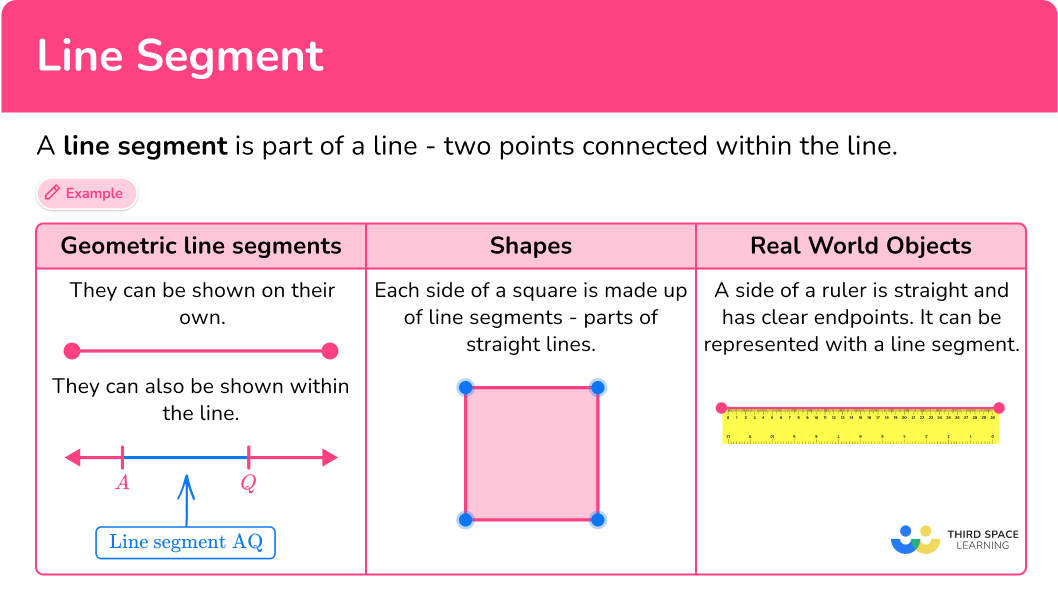## Common Core State Standards

How does this relate to 4 th grade math?

• 4th Grade – Geometry (4.G.A.1)
Draw points, lines, line segments, rays, angles (right, acute, obtuse), and perpendicular and parallel lines. Identify these in two-dimensional figures.

## How to identify line segments

In order to identify line segments:

1. Look for two endpoints.
2. State the name of the line segment(s) using a pair of letters.

## Line segment examples

### Example 1: one dimensional

Is the figure a line segment?

1. Look for two endpoints.

The figure is a line, with arrows pointing in each direction going on forever. There are no endpoints shown.

The figure is not a line segment.

### Example 2: one dimensional

Name a line segment shown.

The figure shows a line, with the points G, H and J. Any of these points can be endpoints of a line segment. Let’s look at all the possibilities.

From point G to H:

From point G to J:

From point H to J:

The line segments are GH, \, GJ and HJ.

### Example 3: line segments in a pentagon

How many line segments are in the pentagon?

Each vertex can be an endpoint. Let’s look at all the possibilities.

The five sides of a pentagon are the 5 line segments AB, BC, CD, DE and AE.

### Example 4: lines in a rhombus

How many line segments are in the rhombus?

Each vertex can be an endpoint. Let’s look at all the possibilities.

The four sides of the rhombus are the 4 line segments WX, XY, YZ and WZ.

### Example 5: lines in the real world

Find a line segment on the cereal box.

There are many endpoints. An example is:

The top edge of the box has two endpoints.

The edge connected by the points is straight, which means they fall on a line.

The picture below shows all the other line segments:

The line segments are AB, BC, CD, AD, AE, BF, CG, DH, EF, FG, GH, EH.

### Example 6: measuring with line segments

Given line segment BE, point R is the midpoint. BR measures 44 \, cm. What is the length of the line segment BE?

There are 3 points that can be endpoints. BE is the longest line segment shown. A midpoint divides a line segment into two equal parts, so

\text { line segment } B R=\text { line segment } R E.

Since \text { line segment } B R=\text { line segment } R E, \text { line segment } R E=44.

The length of the BR and RE can be added to find the length of line segment BE: 44+44=88.

The length of line segment BE is 88 \, cm.

### Teaching tips for line segments

• Give students plenty of opportunities to look for and identify line segments in a variety of places. Worksheets that have a mixture of 2D shapes or real world examples can be useful for this skill, along with physical 2D and 3D objects found in the classroom.

• Encourage students to go beyond just rotely solving practice problems to also creating line segments or requiring them to explain why they classified a figure as a line segment.

### Easy mistakes to make

• Confusing lines and line segments
Line segments have definite endpoints, whereas lines go on forever in opposite directions. This can be confusing, especially since it is impossible to draw a complete line, since they are never ending. This is why it is important to draw lines with arrows on each end and clearly label line segments with two points.

• Thinking that line segments have to be vertical or horizontal
Line segments, just like lines, can be any orientation. As long as a figure has two endpoints and is part of a line, it is a line segment.
For example,
All the figures below are line segments.

• Thinking that line segments have to be a certain length
As long as a figure has two endpoints and is part of a line, it is a line segment, no matter how short or long.
For example,
The dime and the sailboat both have line segments.

### Practice line segment questions

1. Which figure shows line segment AB?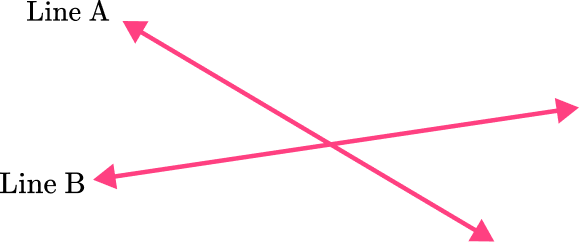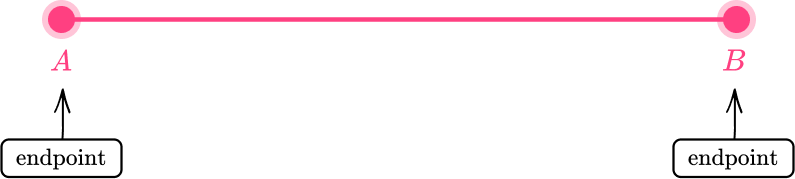Based on the line segment definition, this figure has two clear endpoints. The points are connected by part of a straight line, so it is a line segment.

2. Does connecting points A and B form a line segment? Why or why not?No, because the ends of the line go on forever.Yes, because the ends of the line go on forever.No, because it is two points connected within a line.Yes, because it is two points connected within a line.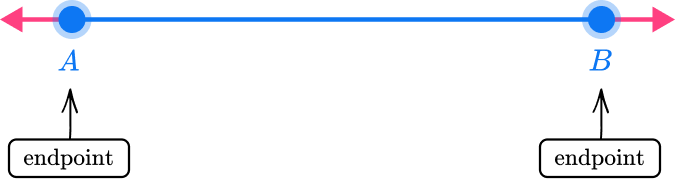This figure has two clear endpoints. The points are connected by part of a straight line, so it is a line segment.

3. Which shape is made up of exactly 4 line segments?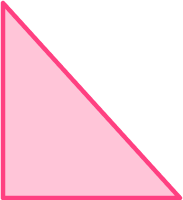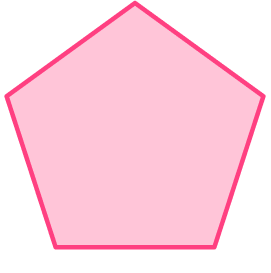Each vertex can be an endpoint. Let’s look at all the possibilities.Each of the sides connected by the points is straight, which means they fall on a line.

The four sides of a trapezoid are 4 line segments.

4. Which shape has exactly 2 line segments?Each vertex can be an endpoint. Let’s look at all the possibilities.This shape has 2 line segments. The curved part of the shape is NOT a line segment, since it is not part of a straight line.

5. Which real world shape (shown in blue) best represents a line segment?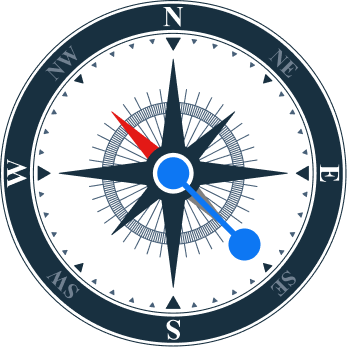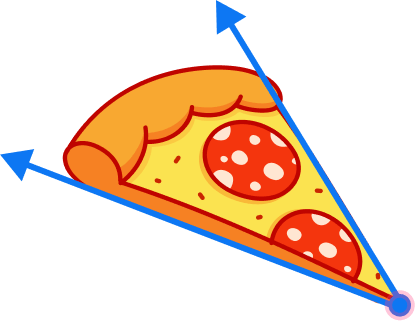The pointer of the compass is marked with clear endpoints that are connected within a straight line. The pointer of the compass represents a line segment.

6. Given line segment TB, point C is the midpoint. TB measures 34 \, inches. What is the length of the line segment TC?17 \, inches64 \, inches34 \, inches12 \, inchesA midpoint divides a line segment into two equal parts. The length of a line segment can be divided by 2 to find the length of the line segment created by a midpoint: 34 \div 2=17.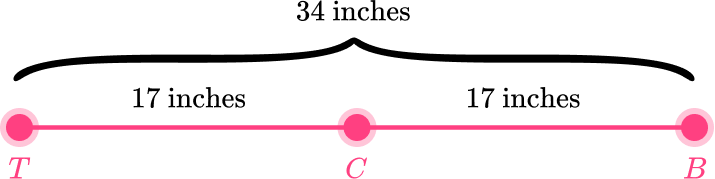The length of line segment TC is 17 \, inches.

## Perpendicular lines FAQs

How many points are there within a line segment?

While two points define the ends of the line segment, the part of the line within the line segment is made up of a collection of points. Since rational numbers can be infinitely small, there are an infinite number of points on any given line segment.

What is the distance formula?

When measuring line segments on a coordinate plane, the distance formula calculates the total distance of the line segment (between the two points).

## Still stuck?

At Third Space Learning, we specialize in helping teachers and school leaders to provide personalized math support for more of their students through high-quality, online one-on-one math tutoring delivered by subject experts.

Each week, our tutors support thousands of students who are at risk of not meeting their grade-level expectations, and help accelerate their progress and boost their confidence.